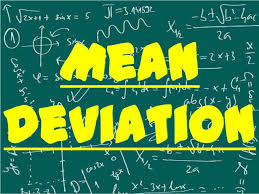# Mean Deviation through MeanNOTE: The given program is only useful for discrete values of X(not continuous and not individual).But can be changed for continuous and individual series.

The following program is written in python.

x = input("Enter the list of numbers(i.e. Value of X) separated by a space : ").split(' ')
f = input("Enter the list of frequencies of the above value of X respectively : ").split(' ')

i = 0
for each_elements in x:
x[i] = int(each_elements)
i += 1
i = 0
for each_elements in f:
f[i] = int(each_elements)
i += 1
i = 0
fx =[]
efx = 0
N = 0
while (i < len(x)) or (i < len(f)):
fx_value = x[i] * f[i]
fx.append(fx_value)
efx = efx + fx[i]
N += f[i]
i += 1
else:
i = 0

mean = efx/N
print(f'The mean of the above data is {mean}..')

individual_deviation = []
for times_of_substraction in range(0,len(x)):
x_minus_mean = x[i] - mean
if '-' in str(x_minus_mean):
x_minus_mean = - x_minus_mean
individual_deviation.append(x_minus_mean)
i += 1
else:
i = 0
final_summation = 0
for time_of_multiplication in range(0,len(individual_deviation)):
y = f[i] * individual_deviation[i]
final_summation += y
i += 1

standard_deviation = final_summation/N
print(f'The standard deviation of the above data is {standard_deviation}')